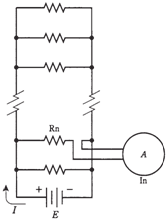## Currents through parallel resistances Assignment Help

Assignment Help: >> Electrical Engineering - Currents through parallel resistances

Currents through parallel resistances

The resistors are called as Rn. The total parallel resistance in circuit is R. The battery voltage is E. The current in the branch n, containing resistance Rn, can be measured by ammeter A and is called as In.Figure: analysis of a current in a parallel circuit.

The sum of all In's in circuit is equal to total current, I, drawn from the source. That is, the current can be divided up in the parallel circuit, similarly to the way that voltage can be divided up in a series circuit.

If you are astute, you will notice that the direction of current flow in the Figure is out from the positive battery terminal. But don't the electrons flow out of the minus terminal? Yes- but scientists consider the current to flow from plus to minus. This is the example of a mathematical convention or "custom." This type of things often outlasts their appropriateness. Physicists had to choose a direction for the flow of current, and plus-to-minus appeared more logical than minus-to-plus. The exact nature of electric current flow was not known then. This notation has not been changed. It was feared that tampering with it would cause confusion only; some people would acknowledge the change while others would not. This might lead to motors running the wrong way, magnets repelling when they should get attracted to each other, transistors being blown out, and other horrors. Look at the mess caused by the conflict between miles and kilometers or between Fahrenheit and Celsius temperatures.

Problem: 1

Suppose that the battery in the given figure delivers 12 V. Further suppose that there are 12 resistors, each having a value of 120 Ω in the parallel circuit. What is total current, I, which is drawn from battery?

First, find total resistance. This is easy, because all resistors have the same value. Simply divide Rn=120 by 12 to get R=10Ω. Then current, I, is found by Ohm's Law: I=E/R=12/10 = 1.2 A.